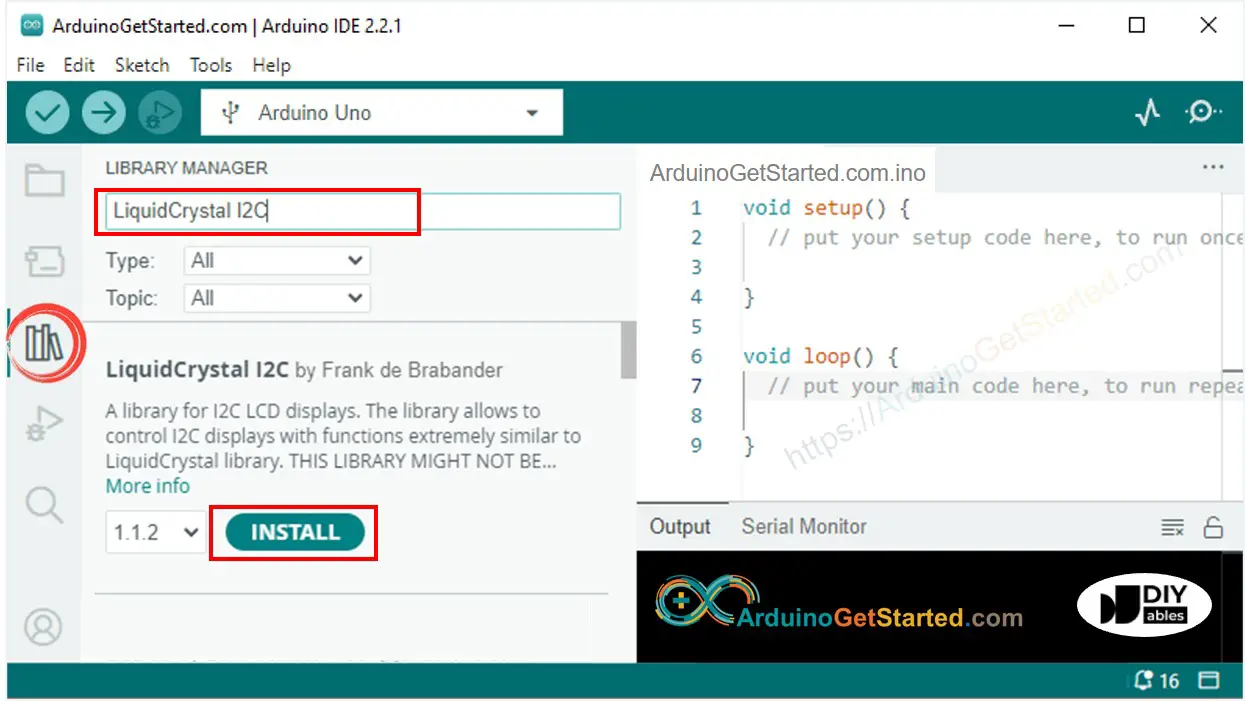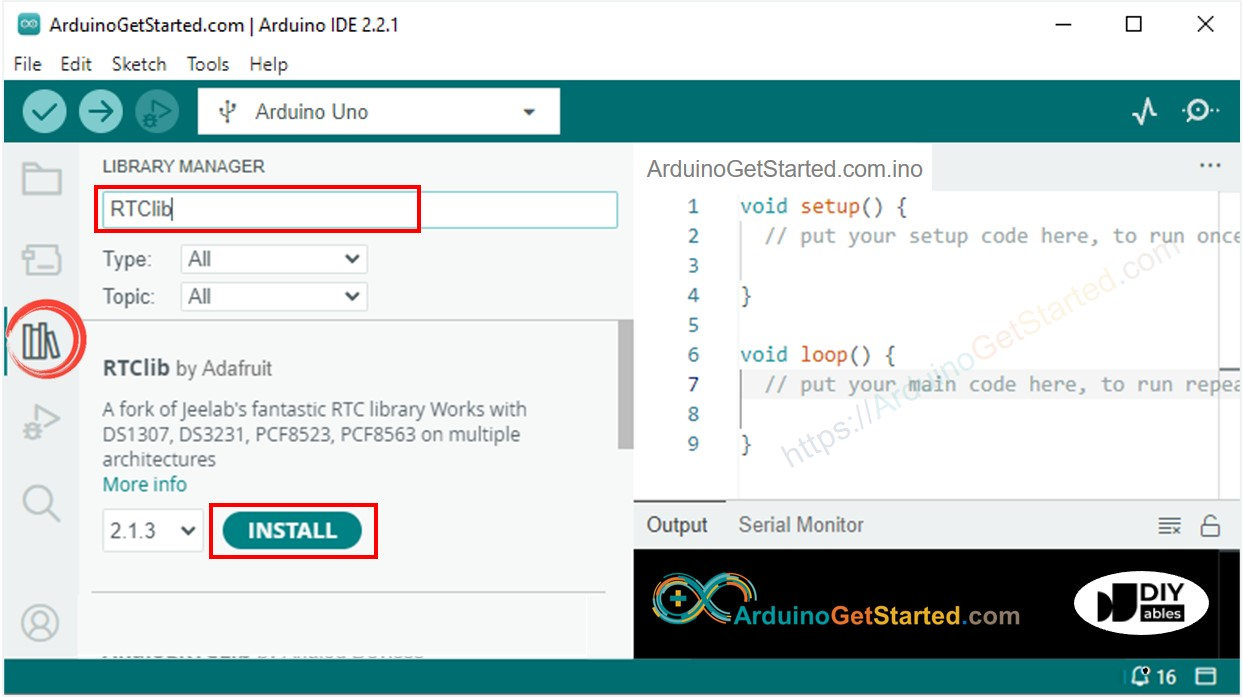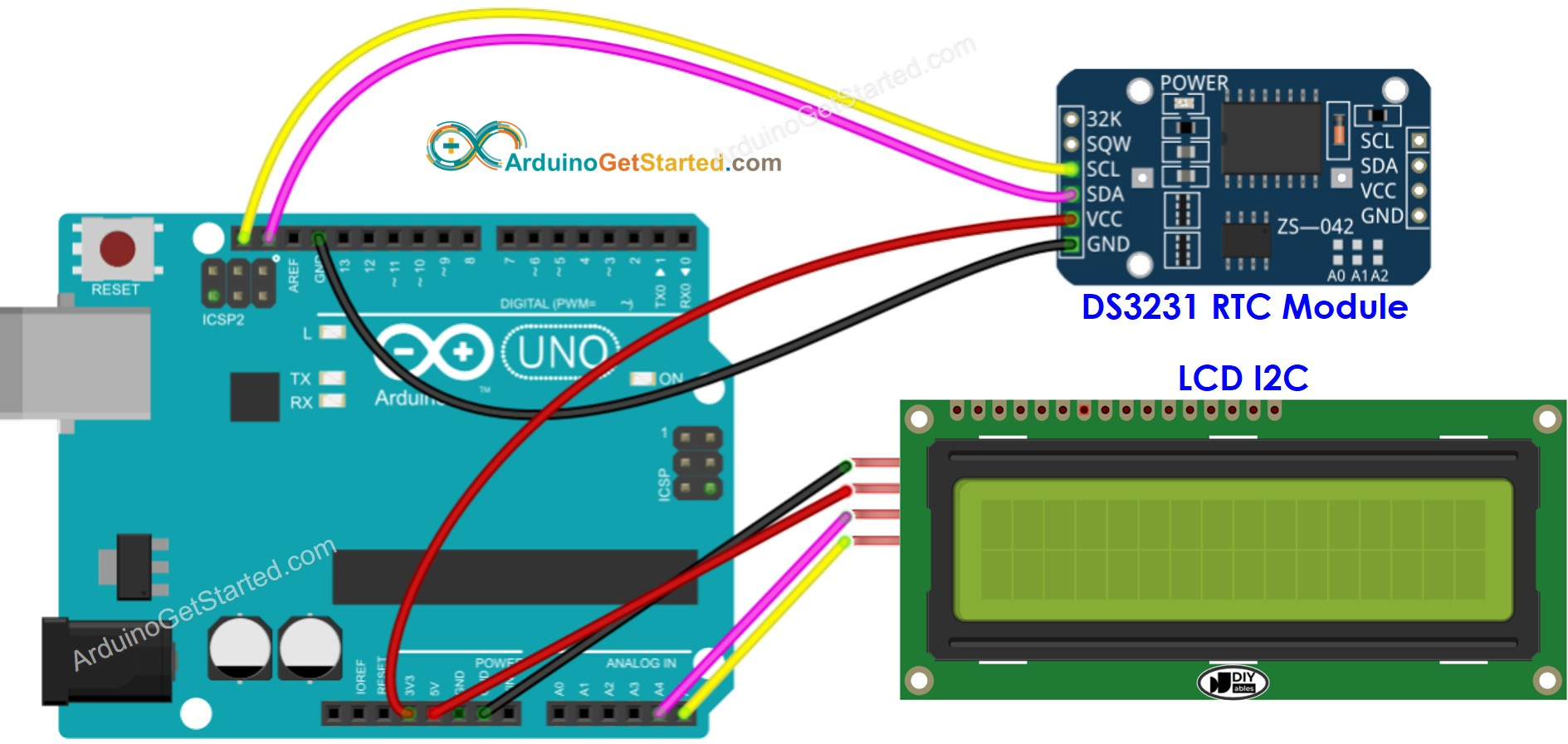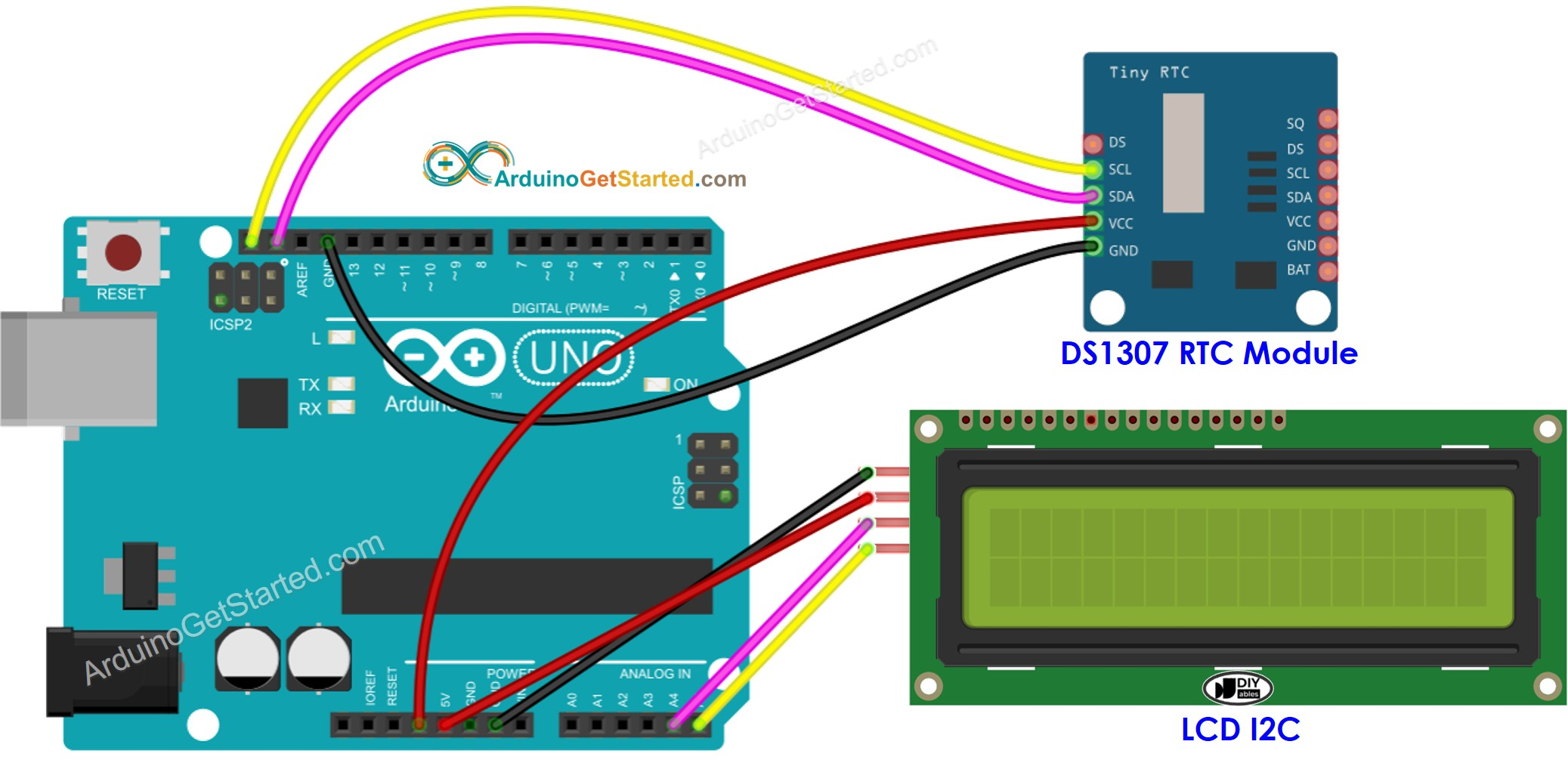# Arduino - LCD Clock

In this tutorial, we are going to learn how to make LCD clock by:

• Reading date and time from DS3231 RTC module and display it on an LCD I2C 16x2
• Reading date and time from DS1307 RTC module and display it on an LCD I2C 16x2

You can choose one of two RTC modules: DS3231 and DS1307. See DS3231 vs DS1307

## Hardware Required

Or you can buy the following sensor kit:

Please note: These are affiliate links. If you buy the components through these links, We may get a commission at no extra cost to you. We appreciate it.

## About LCD, DS3231 and DS1307 RTC module

If you do not know about LCD, DS3231 and DS1307 (pinout, how it works, how to program ...), learn about them in the following tutorials:

## Install LCD and RTC Libraries

• On Arduino IDE, Go to Tools Manage Libraries• Search “LiquidCrystal I2C”, then find the LiquidCrystal_I2C library by Frank de Brabander
• Click Install button to install LiquidCrystal_I2C library.• Search “RTClib”, then find the RTC library by Adafruit. This library works with both DS3231 and DS1307
• Click Install button to install RTC library.## Reading time from DS3231 RTC module and display it on LCD

### Wiring DiagramThis image is created using Fritzing. Click to enlarge image

### Arduino Code - DS3231 and LCD

/* * Created by ArduinoGetStarted.com * * This example code is in the public domain * * Tutorial page: https://arduinogetstarted.com/tutorials/arduino-lcd-clock */ #include <LiquidCrystal_I2C.h> #include <RTClib.h> LiquidCrystal_I2C lcd(0x27, 16, 2); // I2C address 0x3F, 16 column and 2 rows RTC_DS3231 rtc; void setup() { Serial.begin(9600); lcd.init(); // initialize the lcd lcd.backlight(); // open the backlight // SETUP RTC MODULE if (!rtc.begin()) { Serial.println("Couldn't find RTC"); Serial.flush(); while (true) ; } // automatically sets the RTC to the date & time on PC this sketch was compiled rtc.adjust(DateTime(F(__DATE__), F(__TIME__))); } void loop() { DateTime now = rtc.now(); int year = now.year(); int month = now.month(); int day = now.day(); int hour = now.hour(); int minute = now.minute(); int second = now.second(); lcd.clear(); lcd.setCursor(0, 0); // start to print at the first row lcd.print("Date: "); lcd.print(year); lcd.print("/"); lcd.print(month); lcd.print("/"); lcd.print(day); lcd.setCursor(0, 1); // start to print at the second row lcd.print("Time: "); lcd.print(hour); lcd.print(":"); lcd.print(minute); lcd.print(":"); lcd.print(second); delay(1000); // Update every second }

#### Quick Steps

• Copy the above code and open with Arduino IDE
• Click Upload button on Arduino IDE to upload code to Arduino
• See the result on LCD

## Reading time from DS1307 RTC module and display it on LCD

### Wiring DiagramThis image is created using Fritzing. Click to enlarge image

### Arduino Code - DS1307 and LCD

/* * Created by ArduinoGetStarted.com * * This example code is in the public domain * * Tutorial page: https://arduinogetstarted.com/tutorials/arduino-lcd-clock */ #include <LiquidCrystal_I2C.h> #include <RTClib.h> LiquidCrystal_I2C lcd(0x27, 16, 2); // I2C address 0x3F, 16 column and 2 rows RTC_DS1307 rtc; void setup() { Serial.begin(9600); lcd.init(); // initialize the lcd lcd.backlight(); // open the backlight // SETUP RTC MODULE if (!rtc.begin()) { Serial.println("Couldn't find RTC"); Serial.flush(); while (true) ; } // automatically sets the RTC to the date & time on PC this sketch was compiled rtc.adjust(DateTime(F(__DATE__), F(__TIME__))); } void loop() { DateTime now = rtc.now(); int year = now.year(); int month = now.month(); int day = now.day(); int hour = now.hour(); int minute = now.minute(); int second = now.second(); lcd.clear(); lcd.setCursor(0, 0); // start to print at the first row lcd.print("Date: "); lcd.print(year); lcd.print("/"); lcd.print(month); lcd.print("/"); lcd.print(day); lcd.setCursor(0, 1); // start to print at the second row lcd.print("Time: "); lcd.print(hour); lcd.print(":"); lcd.print(minute); lcd.print(":"); lcd.print(second); delay(1000); // Update every second }

#### Quick Steps

• Copy the above code and open with Arduino IDE
• Click Upload button on Arduino IDE to upload code to Arduino
• See the result on LCD

## Video Tutorial

We are considering to make the video tutorials. If you think the video tutorials are essential, please subscribe to our YouTube channel to give us motivation for making the videos.